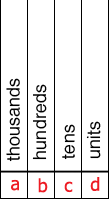SEARCH HOMEMath Central Quandaries & QueriesQuestion from cyndi, a parent: find this 4 digit number: the tens digit is 1/3 of the hundreds digit; the 2 digit number formed by the tens and ones digits i the largest prime number smaller than 40; the sum of all 4 digits is the same as the number of face on an icosahedron? what is the number?Hi Cyndi,

You are to form the four digit number using the digits a, b, c and d is the diagram below.The key here is the two digit number formed from the digits c and d. This is the largest prime number smaller than 40.

Is 39 a prime? No since $39 = 3 \times 13.$

Is 38 a prime? What about 37 and 36 ...?

Now you can find the hundreds digit b.

If you don't know the number of faces on an icosahedron then look it up on the internet.

PennyMath Central is supported by the University of Regina and The Pacific Institute for the Mathematical Sciences.